# Documentation for Graph

We have a very detail documentation for Graph with 8 sections, 23 sub sections, 33 images, 9 paragraphs and 5095 words

## Graph Types

There are many types of graph but in the scope of this course, we will talk about: undirected graphs, directed and weighted graphs

### Undirected Graphs

The edges between any two vertices have no direction, indicating a two-way relationship.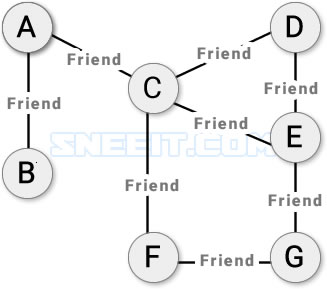Undirected Graph

### Directed Graphs

The edges between any two vertices are directional, indicating a one-way relationship.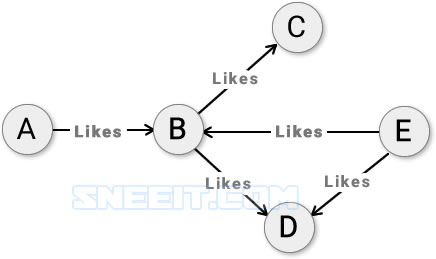Directed Graph

### Weighted Graphs

Each edge has a weight that could represent distance, time, size, etc... The most common "weighted graph" in our daily life is a city map like the below figure where each edge marked with a distance: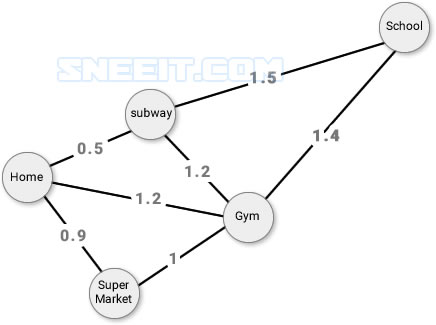Weighted Graph

## Graph Elements

Beside Vertices and Edges, there are some other definitions for other elements in a graph:Figure 2-1

• Path: The sequence of vertices to go through from a vertex to another. Ex: a path from A to C is [A, B, C]. There may be many different paths between two vertices
• Path length: The number of edges in a path. Ex: if a path from A to C is [A,B,C] then its length is 2.
• Cycle: a path where the starting point and ending point is the same vertex. Ex, [I, J, K] is a cycle but [B, E, D] is not
• Negative Weight Cycle: In a "weighted graph", if the sum of all edges in a cycle is negative then that cycle is a negative weight cycle. Ex: [N, Q, S].
• Connectivity: two vertices are connected if there is at least on path between them
• Degree of a Vertex: (unweighted graphs only) is the total direct connections it has. Ex: degree of G is 2
• In-Degree of a Vertex: (directed graphs only) is the number of edges that go into the vertex. In-degree of B is 1 (A into B)
• Out-Degree of a Vertex: (directed graphs only) is the number of edges that go out from the vertex. Out-degree of B is 3 (B out to C, B out to E, B out to D)

## Disjoint Set

### Disjoint Set Overview

Disjoint Set (Union-Find) data structure is a special type of graph where each vertex is a node that has one parent and one or more children.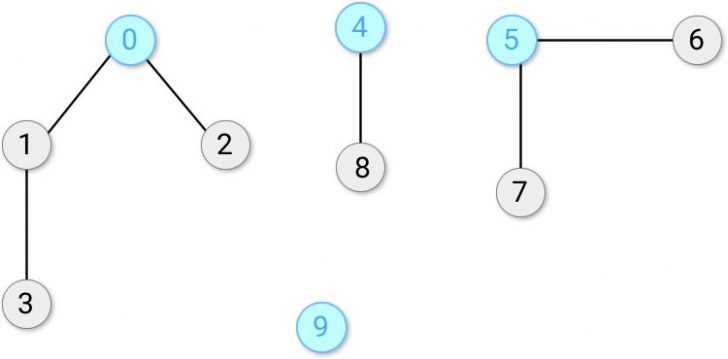Figure 3-1: Disjoint Set

• Parent node: the direct parent node of a vertex. Ex: 0 is the parent of 1 and 2, 4 is the parent of 8, and 9 is the parent of itself.
• Root node (head node): the node that has no parent or it is the parent of itself.

The main purpose of disjoint set is for checking the connectivity of nodes using two functions:

• Find: return the root node of the given nodes
• Union: connect two nodes and make their root nodes the same.

For example: from figure 3-1, we can initialize a root array and perform union and find functions to find the connectivity between some nodes like below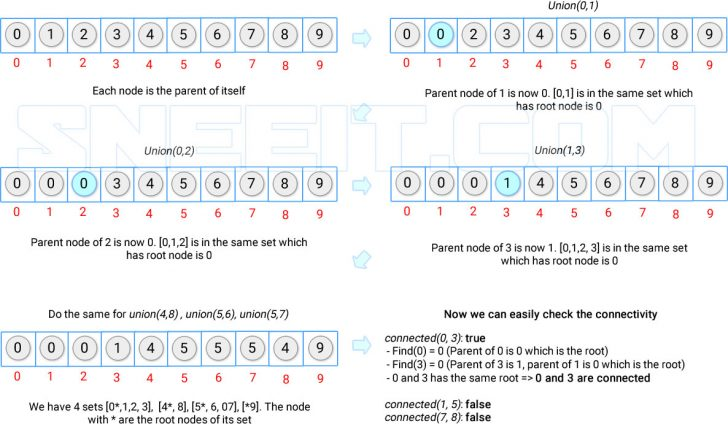Union and Find

In the example above, you can

• union(4, 7) then you should set the parent node of 4 to 7 because 4 was the root node of its set and 7 was not.
• union(4, 5) which are both the root of their current set, then you can set the parent of 4 to 5 or 5 to 4.

### Disjoint Set Quick Find

Quick Find: the values of the root array do not save the parent node of the current node but the real root node instead. This can help find function works quickly but if you want to union two sets, then you need to change the values about the root node for all related nodes.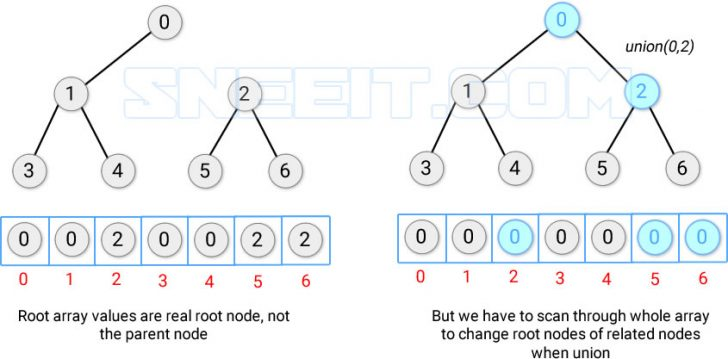Quick Find

The time complexity to union N nodes is O(N^2) because for each node, we may need to go through the whole array to correct the values about the root node.

### Disjoin Set Quick Union

Quick Union: instead of doing union for 2 nodes from two sets, we union the two root nodes of the two sets.Quick Union

The time complexity to union N nodes is also O(N*H) which H is the height of the tree because we need to go through the whole height of tree to find the real root node. Here is the pseudocode

```class DisJoint {
constructor(size) {
this.root = []
for (let i = 0; i < size; i++) {
this.root[i] = i
}
}

find(x) {
if (x == this.root[x])
return x
return this.find(this.root[x])
}

union(x, y) {
let rootX = this.root[x]
let rootY = this.root[y]

if (rootX != rootY) {
this.root[rootY] = this.root[rootX];
}
}

connected(x, y) {
return this.find(x) == this.find(y)
}
}```

### Disjoint Set Quick Union By Rank

If we can keep the height of our tree H < N then we can confidently say that Quick Union is more efficient than Quick Find because O(N* H) < O(N^2) and this is about Quick Union by rank.

To keep the height as smallest as possible, when quick-union, we just need to choose the root node from the higher tree to become the new root node: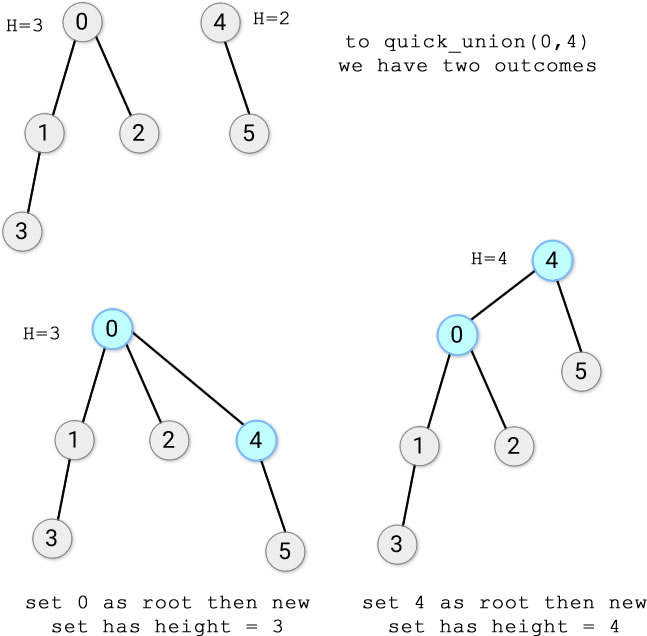Quick Union Options

To do that, we just need to have another array beside the root array to save the rank (represent for the current height of nodes). Here is the pseudocode:

```class DisJoint {
constructor(size) {
this.root = []
this.rank = []
for (let i = 0; i < size; i++) {
this.root[i] = i
this.rank[i] = 1
}
}

find(x) {
if (x == this.root[x])
return x
return this.find(this.root[x])
}

union(x, y) {
let rootX = this.root[x]
let rootY = this.root[y]

if (rootX != rootY) {
if (this.rank[rootX] > this.rank[rootY]) {
this.root[rootY] = this.root[rootX];
} else if (this.rank[rootX] < this.rank[rootY]) {
this.root[rootX] = this.root[rootY];
} else {
this.root[rootY] = this.root[rootX]
this.rank[rootX]++;
}
}
}

connected(x, y) {
return this.find(x) == this.find(y)
}
}```

### DisJoint Set Path Compress

To not repeat the same path again and again when finding the root node for a node, we just need to update the parent node of the current node to the real root node.Compress Path in Quick Union

Here is the pseudocode

```class DisJoint {
constructor(size) {
this.root = []
this.rank = []
for (let i = 0; i < size; i++) {
this.root[i] = i
this.rank[i] = 1
}
}

find(x) {

if (x == this.root[x])
return x
return this.root[x] = this.find(this.root[x])
}

union(x, y) {
let rootX = this.root[x]
let rootY = this.root[y]

if (rootX != rootY) {
if (this.rank[rootX] > this.rank[rootY]) {
this.root[rootY] = this.root[rootX];
} else if (this.rank[rootX] < this.rank[rootY]) {
this.root[rootX] = this.root[rootY];
} else {
this.root[rootY] = this.root[rootX]
this.rank[rootX]++;
}
}
}

connected(x, y) {
return this.find(x) == this.find(y)
}
}```

## Depth-First Search in Graph

Given a graph, how can we find ALL its vertices or all paths between two vertices? This is when we need to use Depth-First Search (DFS) algorithm

### DFS Traversing All Vertices

We can use stack or recursion to traverse / visit all vertices of a graph. The algorithm is to visit a vertex and then visit all of its connected vertices and so on. But we should mark a vertex as a visited vertex if we visited it before so if we go out from that vertex to other vertices, others will not go back to it, which causes an infinity loop.

The following figure will explain about the algorithm of DFS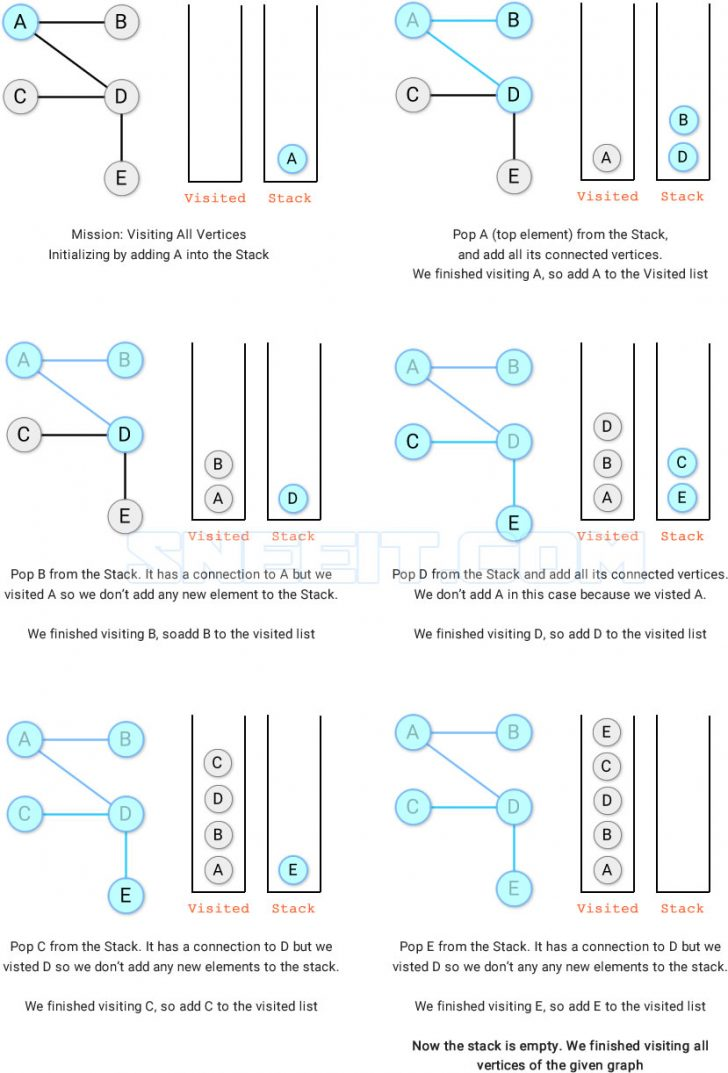DFS Traversing All Vertices

### DFS Find All Paths between Two Vertices

It's similar to traversing all vertices but in this case, we add paths into Stack and manage to undo our paths by removing vertices from Visited.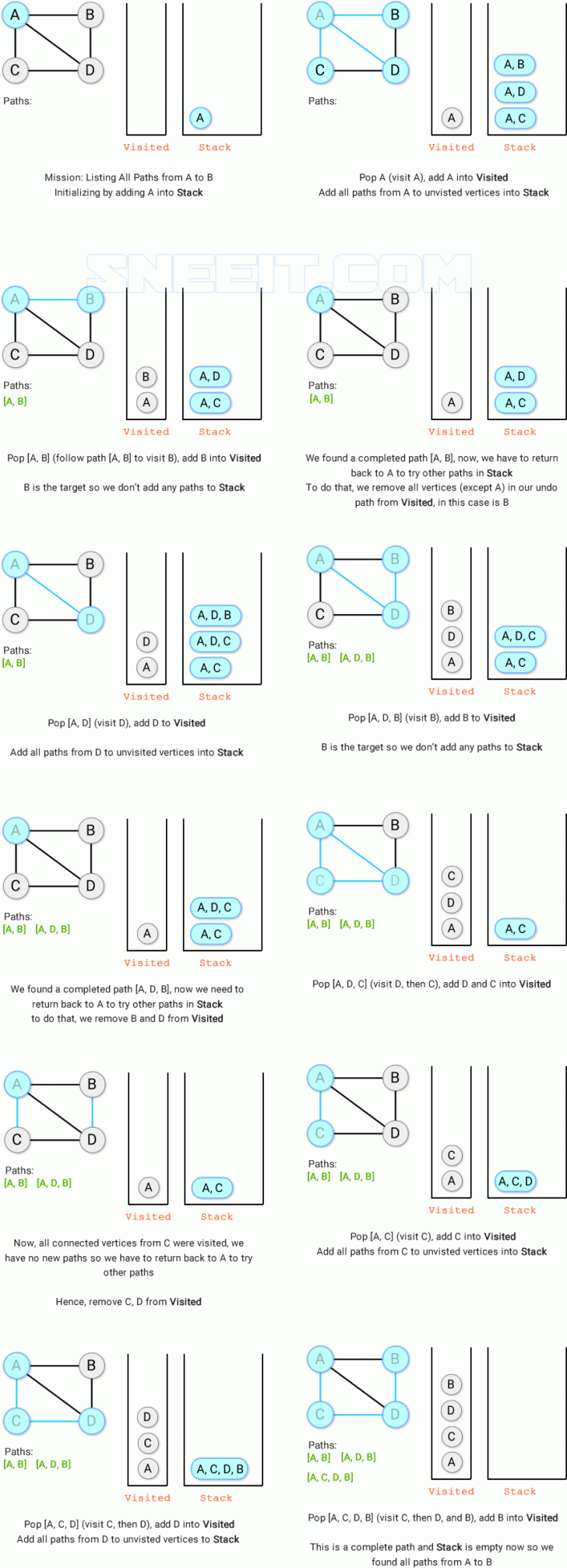DFS - Find All Paths

• Time Complexity is O((V-1)!) because in the worst case when all vertices are connected, there are V -1 available vertices to connect from Source. Then after connecting, the next vertex will will V-2 available vertices to connect which ends up as (v-1) * (v-2) * ... * 2 * 1 = (v-1)!
• Space Complexity is O(V^3) because in the worst case when all vertices are connected, there are V -1 available vertices to connect from Source so Stack has V-1 paths. Then after connecting, the next vertex will will V-2 available vertices to connect so we have to pop Stack and add V-1 paths into Stack which ends up as
(V-1) - 1 + (V-2) - 1 + ... + 1 - 1 = V(V-1)/2 - (V-1) = (V-1) * (V - 2) / 2.
Each paths length may is V so we need space V * (V-1) * (V-2) / 2 = O(V^3)

### DFS Practices

Breadth-First Search (BFS) is like DFS, it can be used to traverse all vertices or all paths between two vertices. But BFS is more efficient in finding the shortest path between two vertices in unweighted graphs (all edges have equal and positive weights). Unlike DFS, BFS does not need to traverse all paths to conclude which path is the shortest one, because as soon as a path is found, it is guaranteed the shortest one.

### BFS Traversing All Vertices

Unlike DFS, BFS uses Queue to traverse level by level to visit all vertices of the given graph.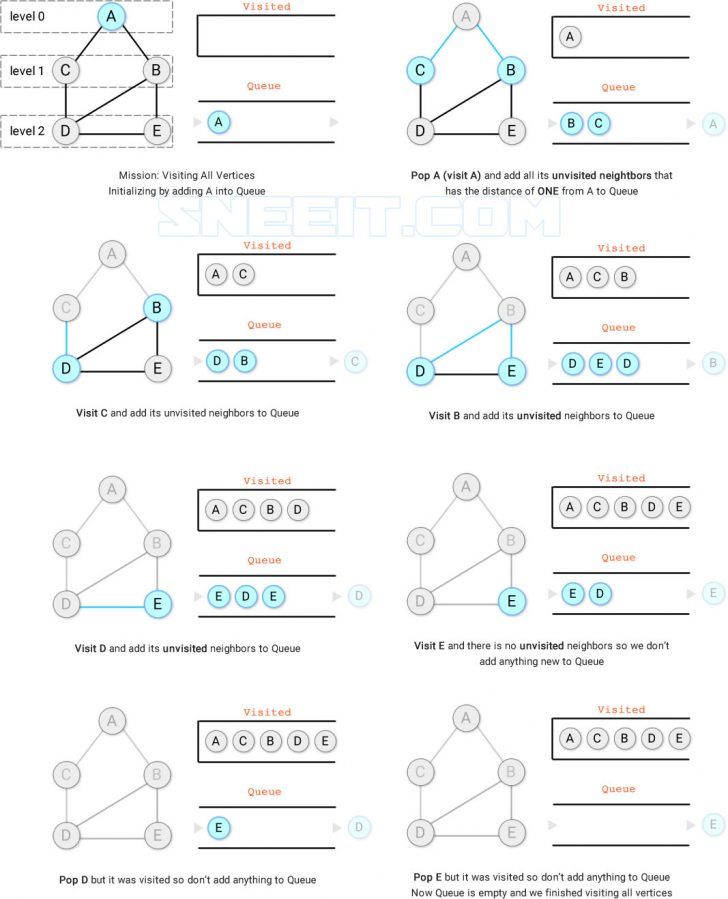BFS - Traverse All Vertices

### BFS Shortest Path Between Vertices

Because we traverse level by level (increasing distance one by one) so the first time we see our target, the time we find the shortest path from source to target.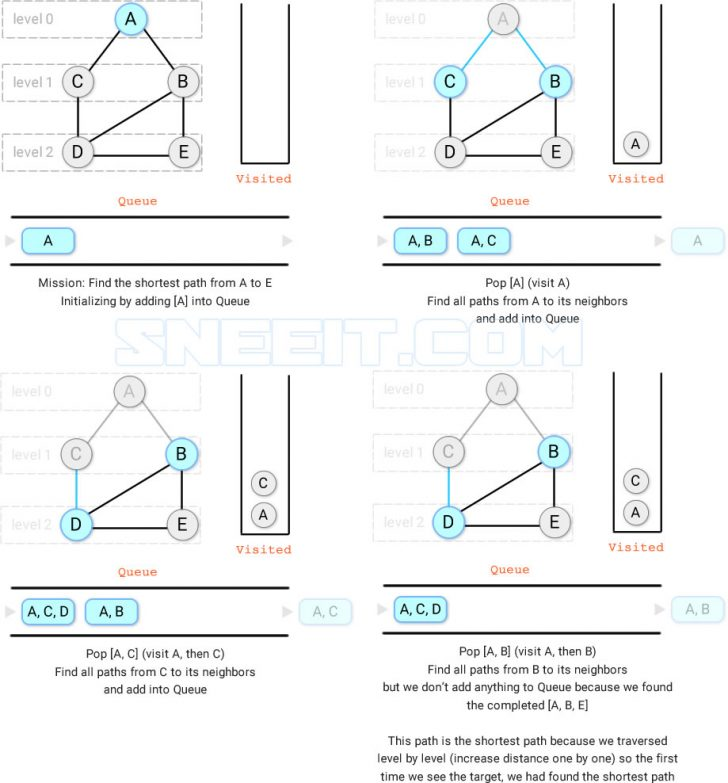BFS - Shortest Path

## Minimum Spanning Tree (MST)

Spanning Tree is a connected subgraph in an undirected graph where all vertices are connected with the number of minimal edges.  In the figure 4.1, the pink edges form a spanning tree of `[A,B], [A,C], [A,D], [A,E]`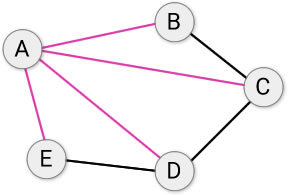Figure 4.1 - Spanning Tree

In figure 4.1, we also have another spanning tree `[A,B], [B,C], [C,D], [D,E]`. Thus, an undirected graph could have more than one spanning tree.

Minimum spanning trees are the ones with the smallest total edge weight in a weighted graph. In Figure 4.2, the green edges form a minimum spanning tree (MST).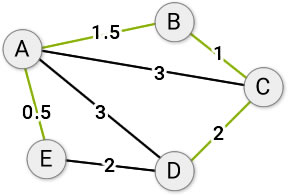Figure 4.2 - Minimum Spanning Tree

A spanning tree has two properties:

1. Has No Cycles
Proof: Assume that a spanning tree T contains a cycle of v1->v2->...->vx->v1. We can remove the edge v1->v2 or vx->v1 and those vertices are still connected or we have a new spanning T' with a smaller number of edges than T. This contracts the definition that a spanning tree has minimal edges.
2. Contain N-1 edges
Proof: every time we want to connect a vertex X to a spanning tree T to form a new spanning tree, we need only one edge (total edges increases by 1), otherwise, we can have a cycle (X->T->X).  So to connect N vertices, we need to increase N-1 times (the first vertex does not need an edge).

### Cut Property

A graph can be split into two disjoint subsets by a "cut" edge and all edges connecting two vertices between two subsets are crossing edges. In figure 4.3, a cut edge splits a graph to two subset s [A,B,E] and [C, D] and all blue edges are crossing edges.Figure 4.3 - Spanning Tree Cut

`"Cut" property: The strictly smallest edge in crossing edges of a cut will belong to all MSTs.`

Proof: Let's say `A` is a crossing edge and it is smaller than ALL crossing edges.

If we have a MST `T` that does not contain A, it should contain another crossing edge `B` (because all vertices are connected in a spanning tree, so there is at least one crossing edge to connect two subsets).

The total weight of T is `X+B` which is the smallest weight to connect all vertices. Now, we replace edge B with edge A then we can have another spanning tree `T'` which has weight `X+A`. Because A is the smallest crossing edge so A < B, or `X+B` < `X+A` or T < T' . This contradicts the assumption that T is a MST.

So A, the smallest crossing edge, will belong to all MSTs

`In other words, if we have two subsets, then the smallest edge that connects the two subsets will belong to ALL MST.`

### Kruskal's Algorithm

Kruskal algorithm could find a MST in a weighted graph using 3 steps:

1. Ascending sort edges by their weight
2. Add edges in that order into a Spanning Tree. Skip the edges that can form a cycle (connect to vertex that is already in the MST)
3. Repeat step 2 until N-1 edges are added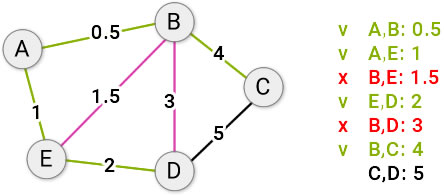Kruscal's Algorithm

Why ascending sort edges by their weight? Due to cut property, we always choose the smallest crossing edge to connect a vertex to a subset. So at a step, the only available smallest edge to connect the current vertex to any subset is E which is larger than all edges in previous subsets (because they used all smallest edges) and smaller than the other edges.

You can practice this algorithm via the problem: Min Cost to Connect All Points

### Prim's Algorithm

Instead of forming multiple spanning trees at a time like Kruskal's algorithm, prim's algorithm maintains only one spanning tree and then adds one by one unvisited vertices to the tree by following the smallest edge from the whole tree to unvisited vertices. We will do this until we have enough N-1 edges in our tree.

Proof: we can cut the graph to two separated subsets at any step: one is our spanning tree, another is the subset for unvisited edges. The only edge that can connect the two subsets is the smallest edge from our spanning tree due to the "cut property". We just need to select the next vertex to connect to our spanning tree following this rule.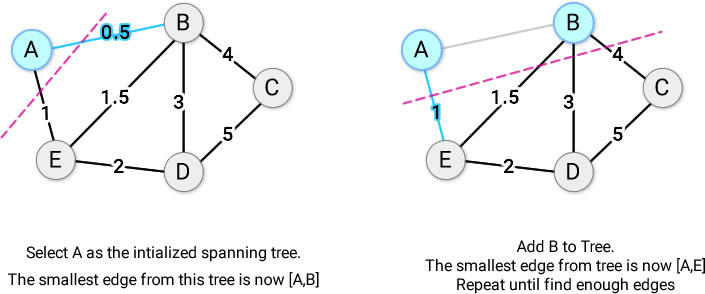Prim's Algorithm

Now you can use Prim's algorithm to solve the same problem: Min Cost to Connect All Points

## Single Source Shortest Path

To find the shortest path from a vertex to any vertices or from a vertex to another in a weighted graph, we cannot use BFS, we need to use other algorithms. But before we learn algorithms, we need to know about edge relaxationFigure 5.1 - Edge Relaxation

Edge relaxation of a vertex is the process to find the shortest distance from the vertex to other vertices. In figure 5.1, A and E are not directly connected so the distance is Infinity. A and D are directly connect with a distance of 3 (like a rubber band stretching 3 times), but there is a shorter distance from A to D if we follow the path [A,C,D] so the distance from A to D is now 2 actually (the rubber band stretching 2 times only so it's relax).

### Dijkstra's Algorithm

Please check the following figure to know about the process of finding shortest paths from a source vertex to all other vertices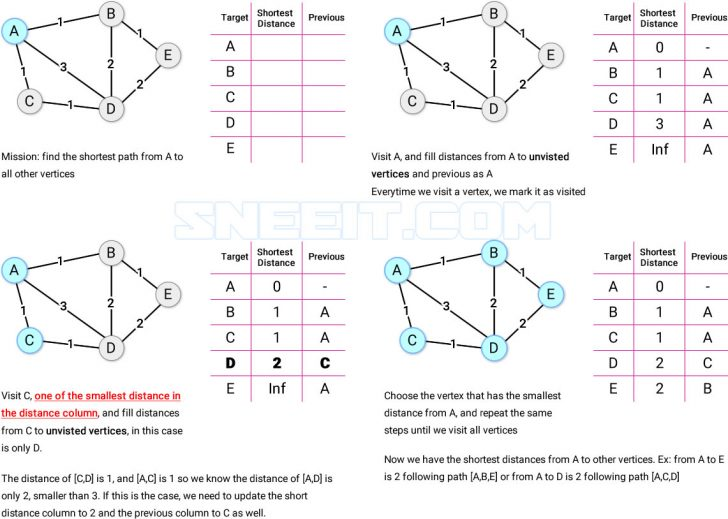Dijkstra's Algorithm

If we add a vertex to the visited list, we will have no chance to recalculate the distance from source to that vertex via unvisited vertices. So if we visit the vertex that has the smallest total distances of nodes back to the source, we assume that value is the smallest distance from source to the vertex.

We have this assumption because all weights of edges are all positive. So if we select another vertex that has a larger distance from source than the current vertex to travel to the current vertex, we only add more distance to the total then the distance from source to the current vertex is still the smallest one.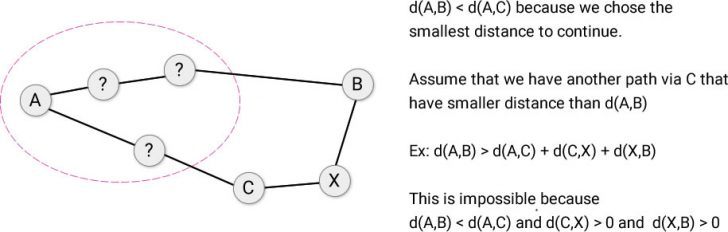Dijkstra's algorithm proof

This assumption is only wrong if we have negative weights because even `d(A,B) < d(A,C)`

we still have a case when d(A,B) > d(A,C) + d(C,X) + D(X,B)

if `d(C,X) + D(X,B)  < d(A,B) - d(A,C)`

or `d(C,X) + D(X,B) < 0`

This is the reason why Dijkstra's algorithm only works with positive weighted graph.

### Bellman Ford's Algorithm

To solve the problem of finding a short path in a weighted graph that has negative weights, we use Bell Ford's Algorithm. This algorithm bases on 2 theorems:

`Theorem 1: in a graph with no negative weighted cycles with N vertices, the shortest path between any two vertices has at most N-1 edges.`

Proof:

• In an acyclic graph (graph with no cycles), the worst case for a path between two vertices is when we have to connect all vertices which is actually a spanning tree where the total edge is N-1.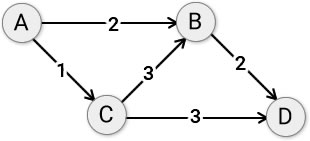• In a no negative weighted cycle graph, we also has only one edge connects two neighbor vertices at a time because if we use more edges to connect the two neighbors, we need to use a cycle, but all cycles are positive weighted so doing that way is only adding up more distance to our path which contradicts the fact that we are finding the shortest path.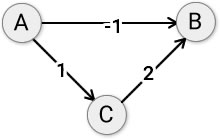`Theorem 2: in a graph with negative weighted cycles there is no shortest path.`

Proof: every time we go through a negative weighted cycle, we will get a smaller distance so we could travel infinitely times to get the -Infinity distance or the shortest path does not exists.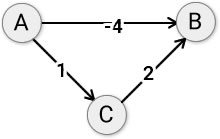#### Bellman Ford's Algorithm Explanation

Because of theorem 1: so our shortest paths may contain 1 to N-1 edges. That's why we could use Dynamic Programming to check all possibilities from 1 to N-1 edges to find the smallest paths from a single source to all other vertices.

The process to calculate the shortest path from A to X in using K edges:

• Find the vertices that are directly connected to X
• For each vertex found (V), the distance from A to X via V equals the distance from A to V using up to K - 1 edges plus the weight of the connection from V to X
`w[A,V,X] = dp[K-1][V] + w[V,X]`
• Then select the minimum values from all calculations to have the shortest path from A to X `d[A,X] or dp[K][X]`

The recurrence relation for our dynamic programming is
`dp[K][X] = min(dp[K-1][V1] + w[V1,X], dp[K-1][V2] + w[V2,X], ...)`

with V1, V2, ... are the vertices that have a direct connection to X.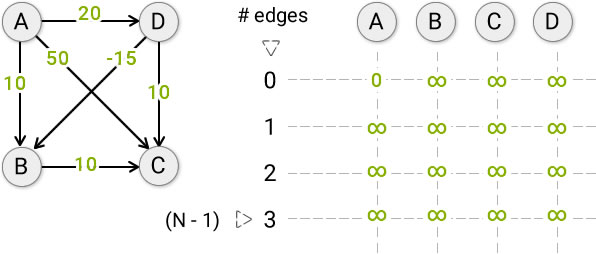Initialization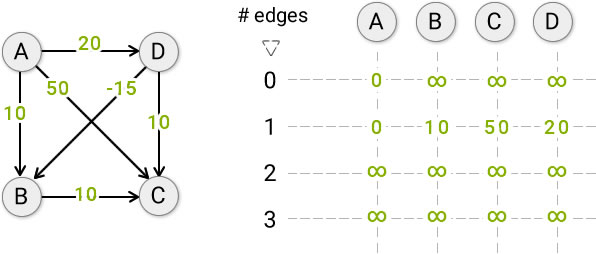To calculate the shortest path from A to B using up to 1 edge

` dp[B] = min(dp[A] + w[A,B], dp[D] + w[D,B])`

= min(0 + 10, ∞ -15) = min(10, ∞) = 10Do the same process and then we will get the final result like above

The number of edges K here is not about finding only the smallest paths that have exactly K edges but any path that can use up to K edges. So dp[K][X] is the min distance from source to X in all paths that can have from 1 up to K edges.

### Bellman Ford's Algorithm Optimizations

#### Optimization 1:

It's clear that we only need the previous row to calculate for the next row in Bellman Ford's Dynamic Programming so instead of using 2D arrays, we just need to use two 1D arrays to store the previous and current values.

#### Optimization 2:

If using the previous array then get the current array that has the same values as the previous array so we can stop because using the same previous array will continue producing the same current array.

#### Optimization 3:

Instead of limiting the number of edges for each iteration, we just imagine that N-1 edges are always allowed, then in each iteration, we scan all outgoing edges from each vertex and update the shortest path from source vertex to the current vertex. We stop our iteration when the previous array equals the current array or we do N-1 iterations.

In case you need to detect if the graph has a negative cycle, do the Nth iteration and then if the current array continue changing (has at least a shorter path updated to the array) so it contradicts the assumption that after N-1 iterations, we should have the shortest paths to all vertices.

This optimization is used only when a problem does not require finding the shortest paths after K stops / edges.

The following figure is the explanation for this optimization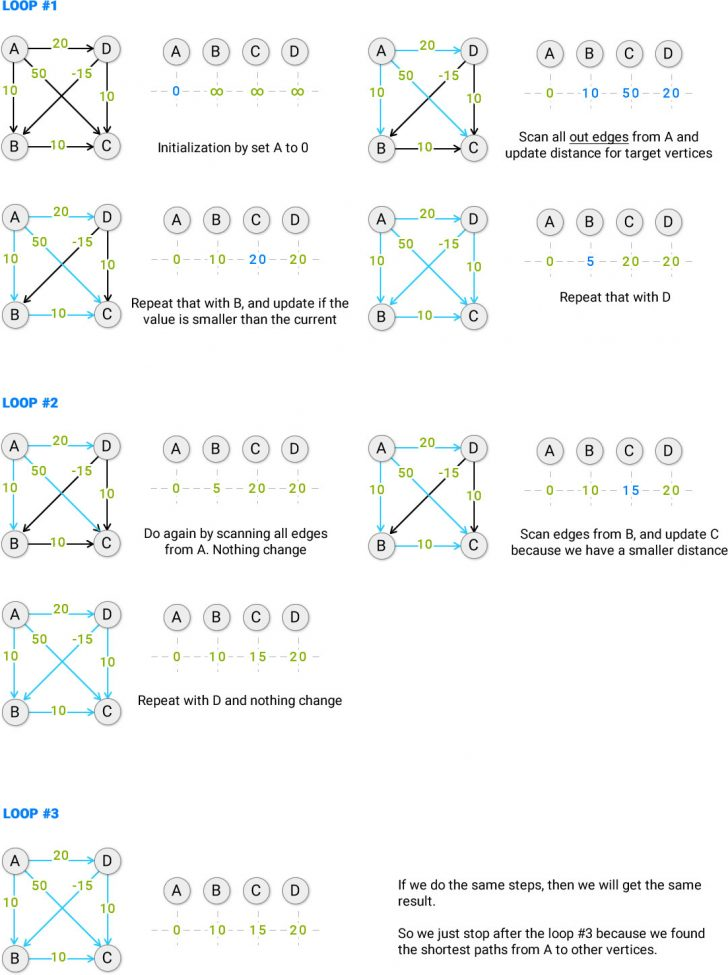Bellman Ford's Algorithm Final

### SPFA Algorithm

If you notice, the order of choosing vertices to scan may affect the number of iterations we need to calculate the final result.Bellman Ford's Algorithm Vertices Order Comparison

So if we can optimize this order, we can find the result earlier, hence, improve the performance of the Bellman Ford's Algorithm.

To do that, we can use the queue to record what are the target vertices that were updated because when a vertex is updated, all paths from it will also be updated as well. If a target vertex has no update, nothing will be affected so we don't need to add it into the queue.

The following figure explains about SPFA (Shortest Paths Faster Algorithm)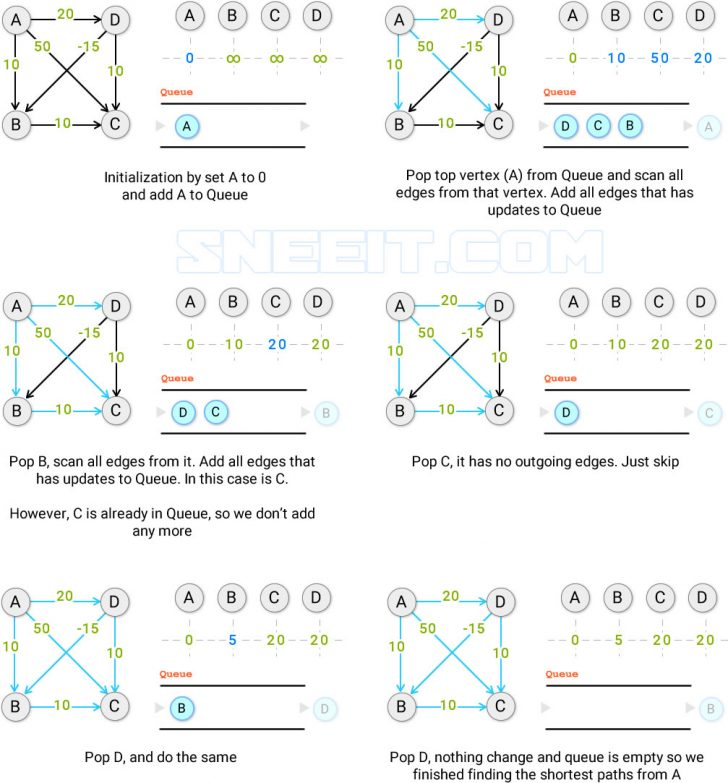SPFA (Shortest Paths Faster Algorithm)

## Kahn's Algorithm for Topological Sorting

If you are given a graph for describing the prerequisite between courses where you have to finish some courses before enrolling in another course. How can we arrange the order of courses following their relationships?Course Relation

To solve that, we use Kahn's Algorithm to perform Topological Sorting. Kahn's Algorithm only works with directed and acyclic graphs that have at least one vertex with ZERO "in-degree".

The following figure explains about the steps in Kahn's AlgorithmKahn's Algorithm

Here are some problems for practicing